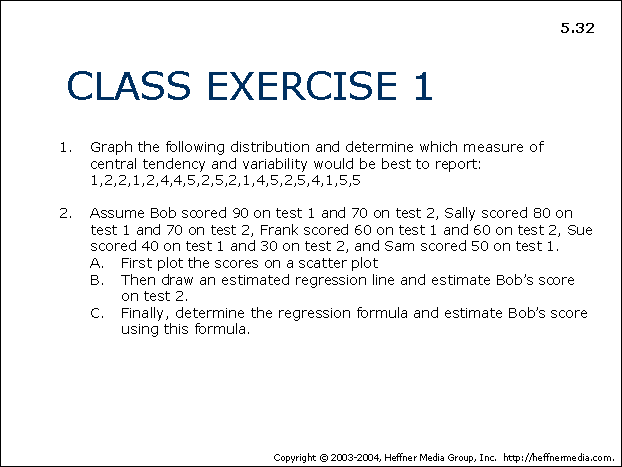# 32: Class Exercise I – Graphing Distribution## CLASS EXERCISE 1

1. Graph the following distribution and determine which measure of central tendency and variability would be best to report:

1,2,2,1,2,4,4,5,2,5,2,1,4,5,2,5,4,1,5,5

1. Assume Bob scored 90 on test 1 and 70 on test 2, Sally scored 80 on test 1 and 70 on test 2, Frank scored 60 on test 1 and 60 on test 2, Sue scored 40 on test 1 and 30 on test 2, and Sam scored 50 on test 1.
• A. First plot the scores on a scatter plot
• B. Then draw an estimated regression line and estimate Bob’s score on test 2.
• C. Finally, determine the regression formula and estimate Bob’s score using this formula.